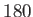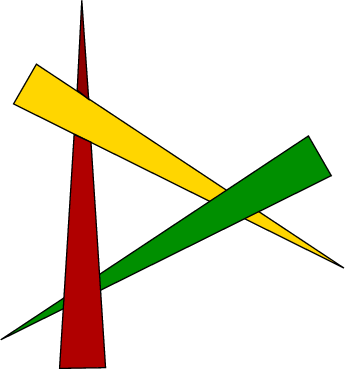# 7.2 Rasterization

The ray casting operation quickly becomes a bottleneck. For a 1080p image at 90Hz, it would need to be performed overmillion times per second, and the ray-triangle intersection test would be performed for every triangle (although data structures such as a BSP would quickly eliminate many from consideration). In most common cases, it is much more efficient to switch from such image-order rendering to object-order rendering. The objects in our case are triangles and the resulting process is called rasterization, which is the main function of modern graphical processing units (GPUs). In this case, an image is rendered by iterating over every triangle and attempting to color the pixels where the triangle lands on the image. The main problem is that the method must solve the unavoidable problem of determining which part, if any, of the triangle is the closest to the focal point (roughly, the location of the virtual eye).One way to solve it is to sort the triangles in depth order so that the closest triangle is last. This enables the triangles to be drawn on the screen in back-to-front order. If they are properly sorted, then any later triangle to be rendered will rightfully clobber the image of previously rendered triangles at the same pixels. The triangles can be drawn one-by-one while totally neglecting the problem of determining which is nearest. This is known as the Painter's algorithm. The main flaw, however, is the potential existence of depth cycles, shown in Figure 7.6, in which three or more triangles cannot be rendered correctly in any order by the Painter's algorithm. One possible fix is to detect such cases and split the triangles.

Subsections
Steven M LaValle 2019-03-14